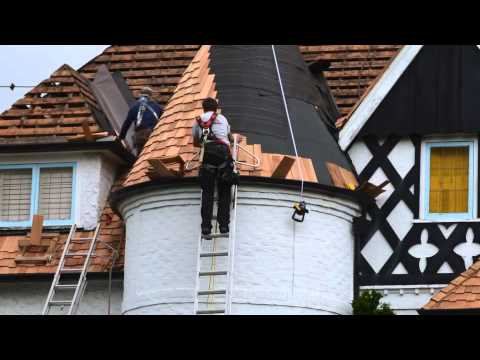## How to Measure the Roof of a Round Building

### How to Measure a Quonset Roof

1. Measure the horizontal width of the Quonset roof to determine the diameter. …
2. Multiply the diameter dimension time 3.14, referred to as “pi,” and then divide the result by 2 to determine the circumference measurement of the Quonset roof.# How to Measure the Roof of a Round Building

1 . Measure the outer diameter of the building.

2 . Measure the amount of horizontal overhang between the building’s outer wall and the roof’s outer edge.

3 . Multiply the amount of overhang by two to include the opposite side of the building.

4 . Add the total overhang to the diameter. For example, a building diameter of 20 feet and an overhang of 1 foot on one side results in a total roof diameter of 22 feet.

5 . Measure the length of the rafters from the peak to the lower edge.

6. Calculate the total surface area of the roof using the following formula: pi times the radius times the rafter length, also called the slant height. To find the surface area using the diameter in the previous example and a rafter length of 20 feet, use the following calculations and use 3.14 as an approximation for pi:

Radius = 22 / 2 = 11.

Surface area in square feet = 3.14 * 11 * 20 = 690.8.

7 .  Determine the vertical height of the roof by finding the square root of the result of subtracting the squares of the rafter length and the radius. Using the previous results, the answer would look like this:

Rafter length squared = 20 * 20 = 400.

Radius squared = 11 * 11 = 121.

So 400 – 121 = 279.

The square root of 279 is approximately 16.7. In this example, the vertical rise of the roof is 16.7 feet from the outer edge to the peak of the roof.# Measuring Roof Slope and Pitch

### Slope

Slope is the incline of the roof expressed as a ratio of the vertical rise to the horizontal run, where the run is some portion of the span. This ratio is always expressed as inches per foot.

### Slope Ratio

A roof that rises 4 inches for every 1 foot or 12 inches of run is said to have a “4 in 12” slope. If the rise is 6 inches for every 12 inches of run, then the roof slope is “6 in 12.”

The slope can be expressed numerically as a ratio. The slope ratio represents a certain amount of vertical rise for every 12 inches of horizontal run. For example, a “4 in 12” slope can be expressed as the ratio of 4:12. A “6 in 12” slope is expressed as 6:12.

### Pitch

Pitch is the incline of the roof expressed as a fraction derived by dividing the rise by the span, where the roof span is the distance between the outside of one wall’s top plate to another.

### Pitch Fraction

Historically the word “pitch” meant a ratio between the ridge height to the entire span/width of the building or ratio between the rafter length to the building width. And back then, the ridge was typically in the middle of the span. This is no longer the case in modern building practices. The ridge can be placed anywhere in the span, from directly middle to either span endpoint.
A roof that rises 8 feet over a 24-foot span was said to have a “1 to 3” pitch. If the rise is 4 feet over a 24-foot span, then the roof pitch was said to be “1 to 6.”

The pitch can be expressed numerically as a fraction. The pitch fraction represents a certain amount of vertical rise over the entire span. For example, given a roof with a rise of 4 feet and a span of 24 feet, the pitch is “1 to 6” pitch, which can be expressed as the fraction of 1/6. A “12 to 24” pitch is expressed as 1/2.
0